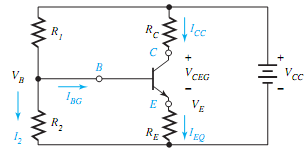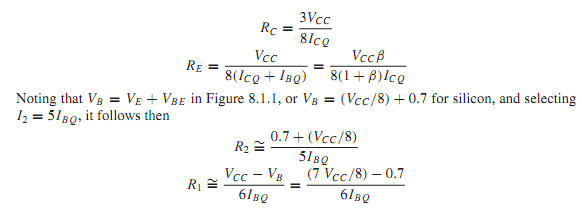## Biasing the bjt, Electrical Engineering

Assignment Help:

Q. Biasing the BJT?

A simple method of biasing the BJT is shown in Figure. While no general biasing procedure that will work in all cases can be outlined, a reasonable approach is to assign 1/2 VCC as the drop across the transistor, 3/8 VCC as the drop across RC to allowan adequate ac voltage swing capability in the collector circuit, and 1/8 VCC as the drop across RE, so that RE is about 1/3 RC. With a speci?ed supply voltage VCC, biasing consists mainly of selecting values for VCEQ, ICQ, and IBQ =  ICQ/β, which de?ne the operating Q point. One can then select#### Digital electronics, In a certain chemical-processing plant, a liquid chemi...

In a certain chemical-processing plant, a liquid chemical is used in a manufacturing process. The chemical is stored in three different tanks. A level sensor in each tank produces

#### Calculate I function using logic gates, I need I circuit that calculates f(...

I need I circuit that calculates f(n) = 2^2^n, where n is a 4bit number. I tried with a decoder but unsuccessfully.

#### What is session layer, Q. What is Session Layer? Session layer is used ...

Q. What is Session Layer? Session layer is used to allow users to identify themselves when waiting access to the network. This is concerned with setting up and sustaining an op

#### Obtain a thevenin equivalent circuit, Q. (a) Obtain a Thevenin equivalen...

Q. (a) Obtain a Thevenin equivalent circuit at terminals A-B in the circuit. (b) What impedance ¯ZL, when connected to A-B, produces maximum power in ¯ZL? (c) Find the val

#### What do you mean by negative impedance converter, Q. What do you mean by Ne...

Q. What do you mean by Negative Impedance Converter? The op-amp circuit of Figure causes a negative resistance R in between the input terminal and ground. In the more general

Vee vcc

#### Calculate terminal voltage, A permanent magnet DC motor is being considered...

A permanent magnet DC motor is being considered for use as a drive motor for a vehicle. The motor is known to have the following properties No load speed @ 100 V = 2000 rpm torq